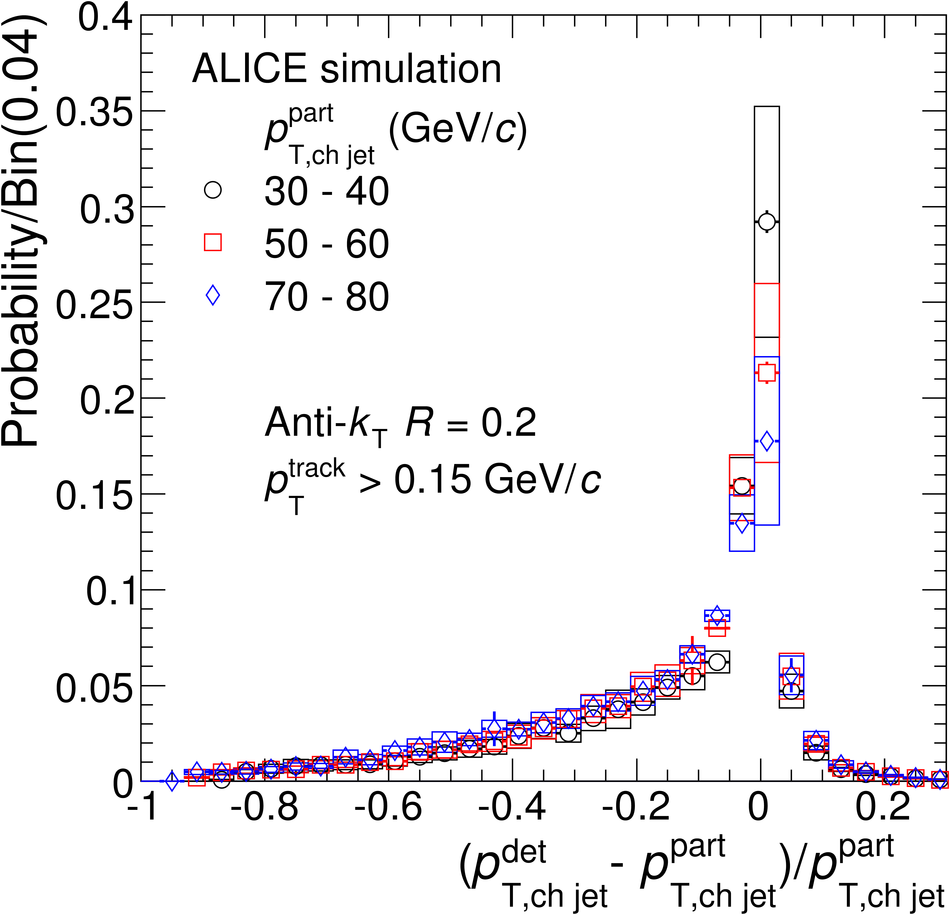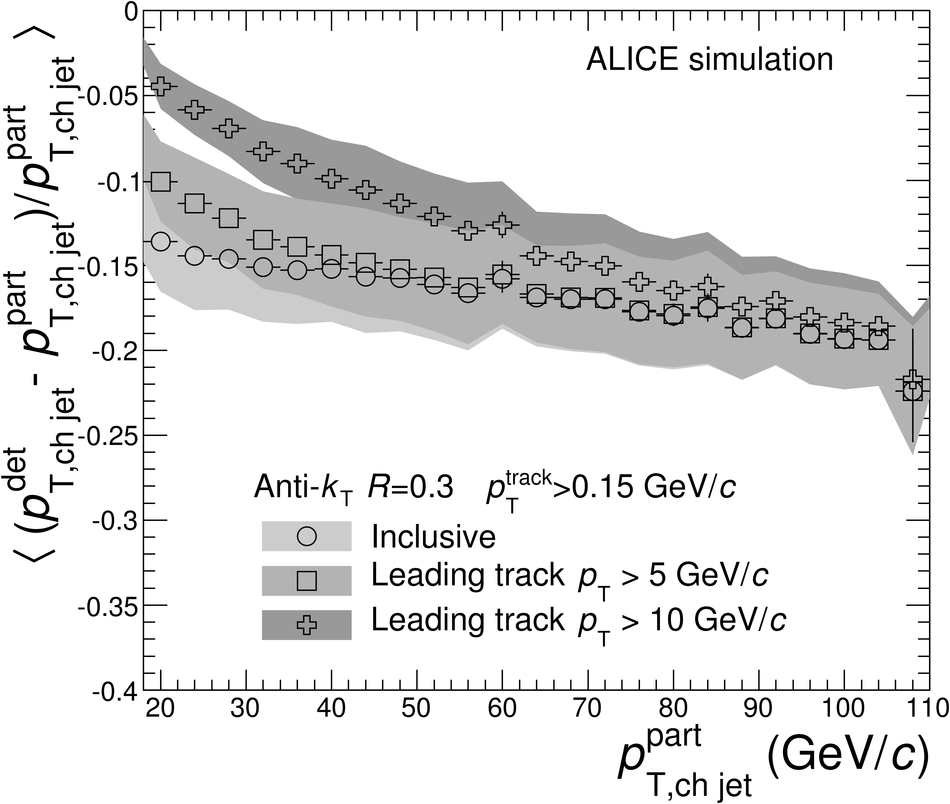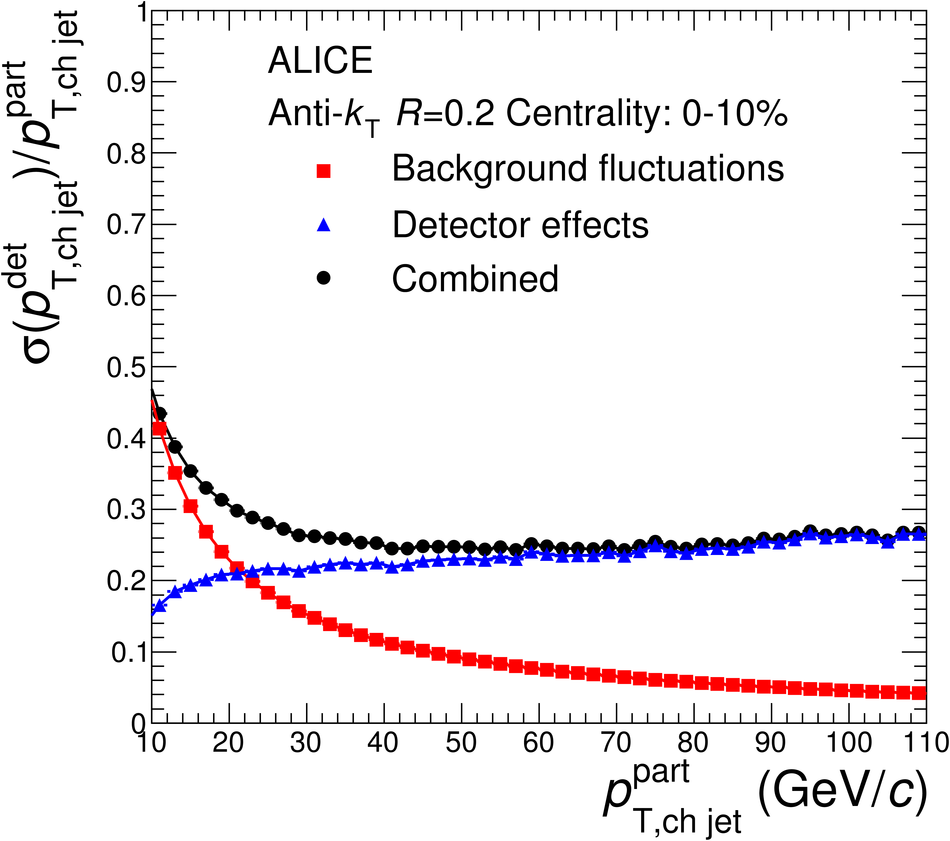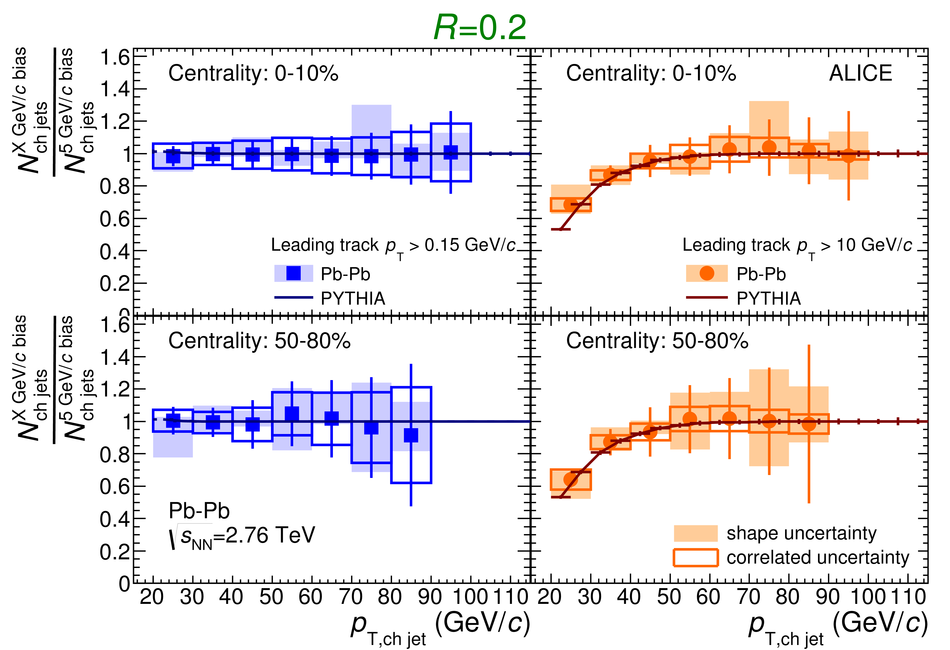# Measurement of charged jet suppression in Pb-Pb collisions at $\sqrt{s_{\rm NN}}=2.76$ TeV

A measurement of the transverse momentum spectra of jets in Pb-Pb collisions at $\sqrt{s_{\rm NN}}=2.76$ TeV is reported. Jets are reconstructed from charged particles using the anti-$k_{\rm T}$ jet algorithm with jet resolution parameters $R$ of $0.2$ and $0.3$ in pseudo-rapidity $|\eta|<0.5$. The transverse momentum $p_{\rm T}$ of charged particles is measured down to $0.15$ GeV/$c$ which gives access to the low $p_{\rm T}$ fragments of the jet. Jets found in heavy-ion collisions are corrected event-by-event for average background density and on an inclusive basis (via unfolding) for residual background fluctuations and detector effects. A strong suppression of jet production in central events with respect to peripheral events is observed. The suppression is found to be similar to the suppression of charged hadrons, which suggests that substantial energy is radiated at angles larger than the jet resolution parameter $R=0.3$ considered in the analysis. The fragmentation bias introduced by selecting jets with a high $p_{\rm T}$ leading particle, which rejects jets with a soft fragmentation pattern, has a similar effect on the jet yield for central and peripheral events. The ratio of jet spectra with $R=0.2$ and $R=0.3$ is found to be similar in Pb-Pb and simulated PYTHIA pp events, indicating no strong broadening of the radial jet structure in the reconstructed jets with $R<0.3$.

Figures

## Figure 1

 Uncorrected jet spectra after background subtraction, with radius parameters $R=0.2$ (top) and $R=0.3$ (bottom) in central Pb-Pb events, without leading particle selection (unbiased, black circles) and with at least one particle with $\pt > 5$ (green crosses) or $10$ GeV/$c$ (red squares).## Figure 2

 Top: $\delta^{\rm ch}_{p_{\rm T}}$ distribution for jets with resolution parameter $R=0.2$ and $R=0.3$ measured with random cones in central collisions. Bottom: Width of the background fluctuation $\delta^{\rm ch}_{p_{\rm T}}$ distribution as a function of centrality for cone radii $R=0.2$ and $R=0.3$. The shaded uncertainty bands indicate the difference between the width of the $\delta_{p^{\rm ch}_{\rm T}}$ distribution from random cones and high-$\pt$ probe embedding.## Figure 3

 Distributions of relative transverse momentum difference between detector and particle level anti-$k_{\rm T}$ jets with $R=0.2$ (top) and $R=0.3$ (bottom) and several ranges of jet transverse momentum at particle level. The distributions correspond to the 10% most central events. Events were generated using PYTHIA with the standard ALICE detector response simulation using GEANT3 and the data reconstruction algorithms and settings used for Pb-Pb events. The dominant systematic uncertainty is the uncertainty on tracking efficiency.## Figure 4

 Jet detector response for jet finding resolution parameter $R=0.3$ for the 10% most central events. Data points extracted from event and full detector simulation. Systematic uncertainty originates from the uncertainty on the tracking efficiency. Top: mean of the jet response for charged jets with $R=0.3$. See text for details. Bottom: Mean, most probably value and quartiles of the jet response as a function of jet momentum.## Figure 5

 Jet-finding efficiency for inclusive unbiased and leading track biased jets extracted from event and detector simulation for the 10% most central events, for $R=0.2$ (top) and $R=0.3$ (bottom).## Figure 6

 Combined jet response for charged jets for the two resolution parameters considered, including background fluctuations and detector effects for 0-10% central Pb-Pb events, for $R=0.2$ (top) and $R=0.3$ (bottom).## Figure 7

 Charged jet spectra, corrected for background fluctuations and detector effects, using two cone radius parameters $R=0.2$ (left panels) and $R=0.3$ (right panels) and different leading track selections: unbiased (top panels), $\pt^{\rm leading}>5$ GeV/$c$ (middle panels), and $\pt^{\rm leading}>10$ GeV/$c$ (bottom panels). The uncertainty bands at $\pt_{\rm jet}^{\rm ch}< 20$ GeV/$c$ indicate the normalisation uncertainty due to the scaling with $1/N_\mathrm{coll}$.## Figure 8

 Ratio of reconstructed unbiased and leading track biased jet yields for two resolution parameters of $R=0.2$ (top) $R=0.3$ (bottom). Calculations of the same ratio with the PYTHIA model (particle level) are shown for reference. Left panels: ratio of unbiased spectra to $\pt^{\rm leading}>5$ GeV/$c$. Right panels: ratio of spectra with $\pt^{\rm leading}>10$ GeV/$c$ to $\pt^{\rm leading}>5$ GeV/$c$.## Figure 9

 Nuclear modification factor $\RCP$ for charged jets with a leading charged particle with $\pT^{\rm leading} >5$ GeV/$c$, with $R=0.2$ (top panel) and $R=0.3$ (bottom panel) and different centrality selections.## Figure 10

 $\RCP$ for unbiased and leading track biased jets with $60< p_{\rm T,chjet} < 70$ GeV/$c$ as a function of the average number of participants in the collision, $R=0.2$ (top) and $R=0.3$ (bottom). For visibility the data points for all jets and for jets with $\pT^{\rm leading} > 10$ GeV/$c$ are shifted to the left and right, respectively.## Figure 11

 Ratio of charged jet $\pT$-spectra with radius parameter $R=0.2$ and $0.3$ and a leading charged particle $\pT^{\rm leading}>5$ GeV/$c$ in PbPb data and simulated PYTHIA events.## Figure 12

 Comparison to jet $RCP$ measured by ATLAS and to charged particle suppression by ALICE and CMS. Note that the underlying parton $\pt$ scale is different at the same measured $\pt$ for charged particles, charged jets and full jets.